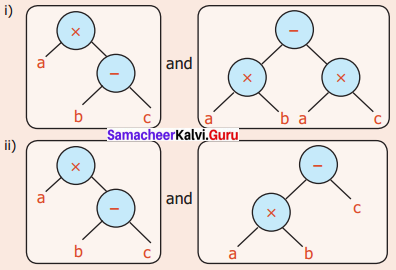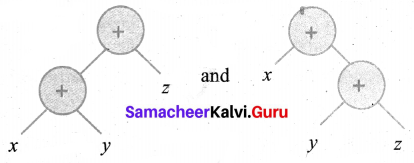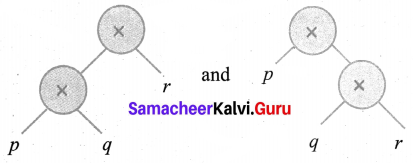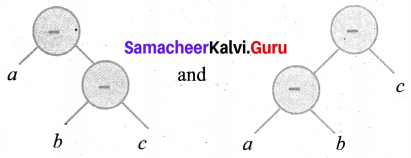# Samacheer Kalvi 6th Maths Solutions Term 2 Chapter 5 Information Processing Intext Questions## Tamilnadu Samacheer Kalvi 6th Maths Solutions Term 2 Chapter 5 Information Processing Intext Questions

Question 1.
Check whether the Tree diagrams are equal or not.Solution:
(i) Their algebraic expressions are a × (b – c) and (a × b) – (a × c)
∴ distributive property of multiplication over subtraction
∴ They are equal
(ii) Their algebraic expressions are a × (b – c) and (a × b) – c
Both are not equal [By BODMAS rule]

Question 2.
Check whether the following algebraic expressions are equal or not by using Tree diagrams.
(i) (x + y) + z and x + (y + z)
(ii) (p × q) × r and p × (q × r)
(iii) a – (b – c) and (a – b) – c
Solution:
(i) (x + y) + z and x + (y + z)
The tree diagrams areSubtraction is not associative and the expressions are not equal.
(ii) (p × q) × r and p × (q × r)
The tree diagram isMultiplication is associative
∴ Both expressions are equal.(iii) a – (b – c) and (a – b) – c
Their tree diagrams areSubtraction is not associative
∴ Both expressions are not equal.